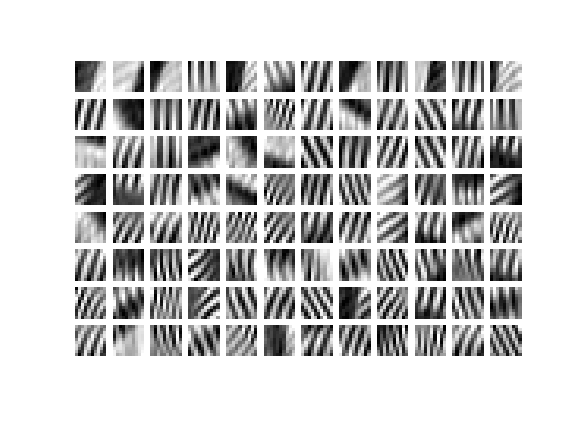# Dictionary Learning¶

Important: Please read the installation page for details about how to install the toolboxes. $\newcommand{\dotp}{\langle #1, #2 \rangle}$ $\newcommand{\enscond}{\lbrace #1, #2 \rbrace}$ $\newcommand{\pd}{ \frac{ \partial #1}{\partial #2} }$ $\newcommand{\umin}{\underset{#1}{\min}\;}$ $\newcommand{\umax}{\underset{#1}{\max}\;}$ $\newcommand{\umin}{\underset{#1}{\min}\;}$ $\newcommand{\uargmin}{\underset{#1}{argmin}\;}$ $\newcommand{\norm}{\|#1\|}$ $\newcommand{\abs}{\left|#1\right|}$ $\newcommand{\choice}{ \left\{ \begin{array}{l} #1 \end{array} \right. }$ $\newcommand{\pa}{\left(#1\right)}$ $\newcommand{\diag}{{diag}\left( #1 \right)}$ $\newcommand{\qandq}{\quad\text{and}\quad}$ $\newcommand{\qwhereq}{\quad\text{where}\quad}$ $\newcommand{\qifq}{ \quad \text{if} \quad }$ $\newcommand{\qarrq}{ \quad \Longrightarrow \quad }$ $\newcommand{\ZZ}{\mathbb{Z}}$ $\newcommand{\CC}{\mathbb{C}}$ $\newcommand{\RR}{\mathbb{R}}$ $\newcommand{\EE}{\mathbb{E}}$ $\newcommand{\Zz}{\mathcal{Z}}$ $\newcommand{\Ww}{\mathcal{W}}$ $\newcommand{\Vv}{\mathcal{V}}$ $\newcommand{\Nn}{\mathcal{N}}$ $\newcommand{\NN}{\mathcal{N}}$ $\newcommand{\Hh}{\mathcal{H}}$ $\newcommand{\Bb}{\mathcal{B}}$ $\newcommand{\Ee}{\mathcal{E}}$ $\newcommand{\Cc}{\mathcal{C}}$ $\newcommand{\Gg}{\mathcal{G}}$ $\newcommand{\Ss}{\mathcal{S}}$ $\newcommand{\Pp}{\mathcal{P}}$ $\newcommand{\Ff}{\mathcal{F}}$ $\newcommand{\Xx}{\mathcal{X}}$ $\newcommand{\Mm}{\mathcal{M}}$ $\newcommand{\Ii}{\mathcal{I}}$ $\newcommand{\Dd}{\mathcal{D}}$ $\newcommand{\Ll}{\mathcal{L}}$ $\newcommand{\Tt}{\mathcal{T}}$ $\newcommand{\si}{\sigma}$ $\newcommand{\al}{\alpha}$ $\newcommand{\la}{\lambda}$ $\newcommand{\ga}{\gamma}$ $\newcommand{\Ga}{\Gamma}$ $\newcommand{\La}{\Lambda}$ $\newcommand{\si}{\sigma}$ $\newcommand{\Si}{\Sigma}$ $\newcommand{\be}{\beta}$ $\newcommand{\de}{\delta}$ $\newcommand{\De}{\Delta}$ $\newcommand{\phi}{\varphi}$ $\newcommand{\th}{\theta}$ $\newcommand{\om}{\omega}$ $\newcommand{\Om}{\Omega}$

Instead of using a fixed data representation such as wavelets or Fourier, one can learn the representation (the dictionary) to optimize the sparsity of the representation for a large class of exemplar.

In :
addpath('toolbox_signal')


## Dictionary Learning as a Non-convex Optimization Problem¶

Given a set $Y = (y_j)_{j=1}^m \in \RR^{n \times m}$ of $m$ signals $y_j \in \RR^m$, dictionary learning aims at finding the best dictionary $D=(d_i)_{i=1}^p$ of $p$ atoms $d_i \in \RR^n$ to sparse code all the data.

In this numerical tour, we consider an application to image denoising, so that each $y_j \in \RR^n$ is a patch of size $n=w \times w$ extracted from the noisy image.

The idea of learning dictionaries to sparse code image patch was first proposed in:

Olshausen BA, and Field DJ., <http://www.nature.com/nature/journal/v381/n6583/abs/381607a0.html Emergence of Simple-Cell Receptive Field Properties by Learning a Sparse Code for Natural Images.> Nature, 381: 607-609, 1996.

The sparse coding of a single data $y=y_j$ for some $j=1,\ldots,m$ is obtained by minimizing a $\ell^0$ constrained optimization $$\umin{ \norm{x}_0 \leq k } \frac{1}{2}\norm{y-Dx}^2 .$$ where the $\ell^0$ pseudo-norm of $x \in \RR^p$ is $$\norm{x}_0 = \abs{\enscond{i}{x(i) \neq 0}}.$$

The parameter $k>0$ controls the amount of sparsity.

Dictionary learning performs an optimization both on the dictionary $D$ and the set of coefficients $X = (x_j)_{j=1}^m \in \RR^{p \times m}$ where, for $j=1,\ldots,m$, $x_j$ is the set of coefficients of the data $y_j$. This joint optimization reads $$\umin{ D \in \Dd, X \in \Xx_k } E(X,D) = \frac{1}{2}\norm{Y-DX}^2 = \frac{1}{2} \sum_{j=1}^m \norm{y_j - D x_j}^2.$$

The constraint set on $D$ reads $$\Dd = \enscond{D \in \RR^{n \times p} }{ \forall i=1,\ldots,p, \quad \norm{D_{\cdot,i}} \leq 1 },$$ (the columns of the dictionary are unit normalized). The sparsity constraint set on $X$ reads $$\Xx_k = \enscond{X \in \RR^{p \times m}}{ \forall j, \: \norm{X_{\cdot,j}}_0 \leq k }.$$

We propose to use a block-coordinate descent method to minimize $E$: $$X^{(\ell+1)} \in \uargmin{X \in \Xx_k} E(X,D^{(\ell)}),$$ $$D^{(\ell+1)} \in \uargmin{D \in \Dd} E(X^{(\ell+1)},D).$$

One can show the convergence of this minimization scheme, see for instance

P. Tseng, <http://www.math.washington.edu/~tseng/papers/archive/bcr_jota.pdf Convergence of Block Coordinate Descent Method for Nondifferentiable Minimization>, J. Optim. Theory Appl., 109, 2001, 475-494.

We now define the parameter of the problem.

Width $w$ of the patches.

In :
if not(exist('w'))
w = 10;
end


Dimension $n= w \times w$ of the data to be sparse coded.

In :
n = w*w;


Number of atoms $p$ in the dictionary.

In :
p = 2*n;


Number $m$ of patches used for the training.

In :
m = 20*p;


Target sparsity $k$.

In :
k = 4;


## Patch Extraction¶

Since the learning is computationnaly intensive, one can only apply it to small patches extracted from an image.

In :
if not(exist('f'))
end
n0 = size(f,1);


Display the input image.

In :
clf;
imageplot(clamp(f));Random patch location.

In :
q = 3*m;
x = floor( rand(1,1,q)*(n0-w) )+1;
y = floor( rand(1,1,q)*(n0-w) )+1;


Extract lots of patches $y_j \in \RR^n$, and store them in a matrix $Y=(y_j)_{j=1}^m$.

In :
[dY,dX] = meshgrid(0:w-1,0:w-1);
Xp = repmat(dX,[1 1 q]) + repmat(x, [w w 1]);
Yp = repmat(dY,[1 1 q]) + repmat(y, [w w 1]);
Y = f(Xp+(Yp-1)*n0);
Y = reshape(Y, [n q]);


We remove the mean, since we are going to learn a dictionary of zero-mean and unit norm atom.

In :
Y = Y - repmat( mean(Y), [n 1] );


Only keep those with largest energy.

In :
[tmp,I] = sort(sum(Y.^2), 'descend');
Y = Y(:,I(1:m));


We consider a dictionary $D \in \RR^{n \times p}$ of $p \geq n$ atoms in $\RR^n$. The initial dictionary $D$ is computed by a random selection of patches, and we normalize them to be unit-norm.

In :
ProjC = @(D)D ./ repmat( sqrt(sum(D.^2)), [w^2, 1] );
sel = randperm(m); sel = sel(1:p);
D0 = ProjC( Y(:,sel) );
D = D0;


Display the initial dictionary.

In :
clf;
plot_dictionnary(D, [], [8 12]);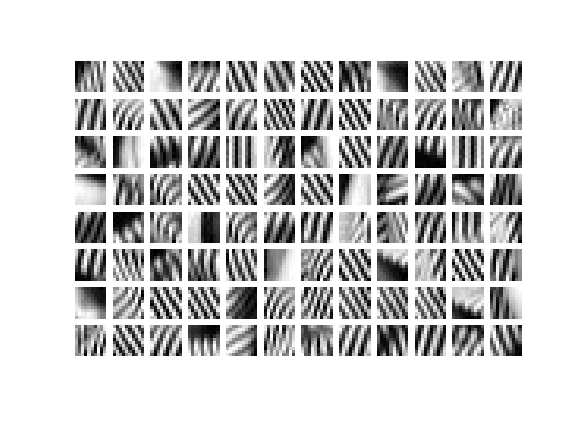## Update of the Coefficients $X$¶

The optimization on the coefficients $X$ requires, for each $y_j = Y_{\cdot,j}$ to compute $x_j = X_{\cdot,j}$ that solves $$\umin{ \norm{x_j}_0 \leq k } \frac{1}{2} \norm{y-D x_j}^2.$$

This is a non-smooth and non-convex minimization, that can be shown to be NP-hard. A heuristic to solve this method is to compute a stationary point of the energy using the Foward-Backward iterative scheme (projected gradient descent): $$x_j \leftarrow \text{Proj}_{\Xx_k}\pa{ x_j - \tau D^* ( D x_j - y ) } \qwhereq \tau < \frac{2}{\norm{D D^*}}.$$

Denoting $\abs{\bar x(1)} \leq \ldots \leq \abs{\bar x(n)}$ the ordered magnitudes of a vector $x \in \RR^n$, the orthogonal projector on $\Xx_k$ reads $z = \text{Proj}_{\Xx_k}(x)$ with $$\forall i=1,\ldots,n, \quad z(i) = \choice{ x(i) \qifq \abs{x(i)} \geq \abs{\bar x(k)}, \\ z(i) = 0 \quad \text{otherwise}. }$$

In :
select = @(A,k)repmat(A(k,:), [size(A,1) 1]);
ProjX = @(X,k)X .* (abs(X) >= select(sort(abs(X), 'descend'),k));


Exercise 1

Perform the iterative hard thresholding, and display the decay of the energy $J(x_j) = \norm{y_j-D x_j}^2$ for several $j$. Remark: note that the iteration can be performed in parallel on all $x_j$.

In :
exo1()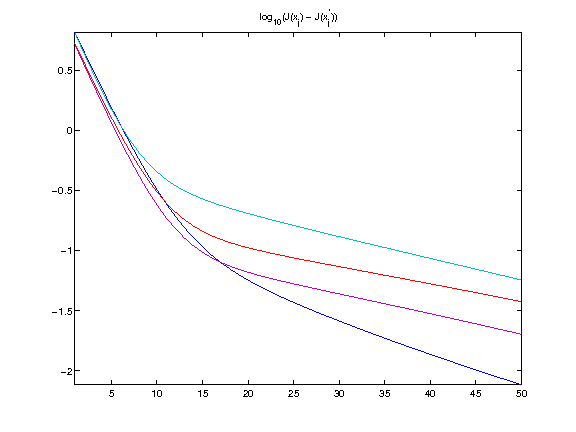In :
%% Insert your code here.


## Update the Dictionary $D$¶

Once the sparse coefficients $X$ have been computed, one can udpate the dictionary. This is achieve by performing the minimization $$\umin{D \in \Dd} \frac{1}{2}\norm{Y-D X}^2.$$

One can perform this minimization with a projected gradient descent $$D \leftarrow \text{Proj}_{\Cc}\pa{ D - \tau (DX - Y)X^* }$$ where $\tau < 2/\norm{XX^*}.$

Note that the orthogonal projector $\text{Proj}_{\Cc}$ is implemented in the function |ProjC| already defined.

Exercise 2

Perform this gradient descent, and monitor the decay of the energy.

In :
exo2()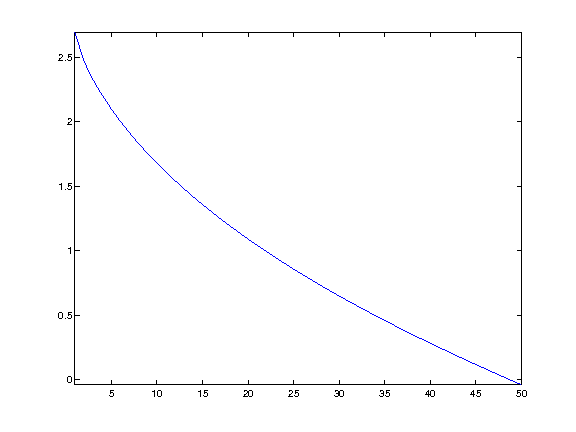In :
%% Insert your code here.


Exercise 3

Perform the dictionary learning by iterating between sparse coding and dictionary update.

In :
exo3()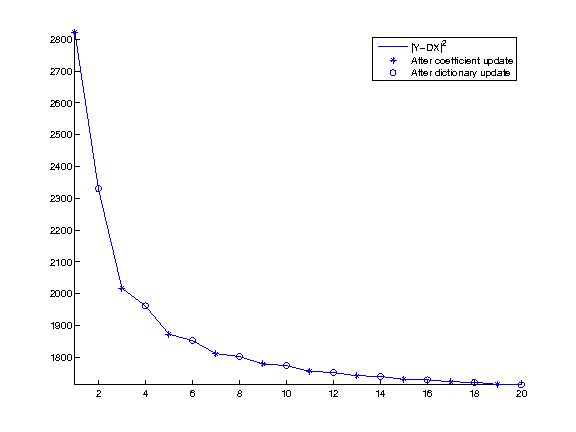In :
%% Insert your code here.


Display the dictionary.

In :
clf;
plot_dictionnary(D,X, [8 12]);